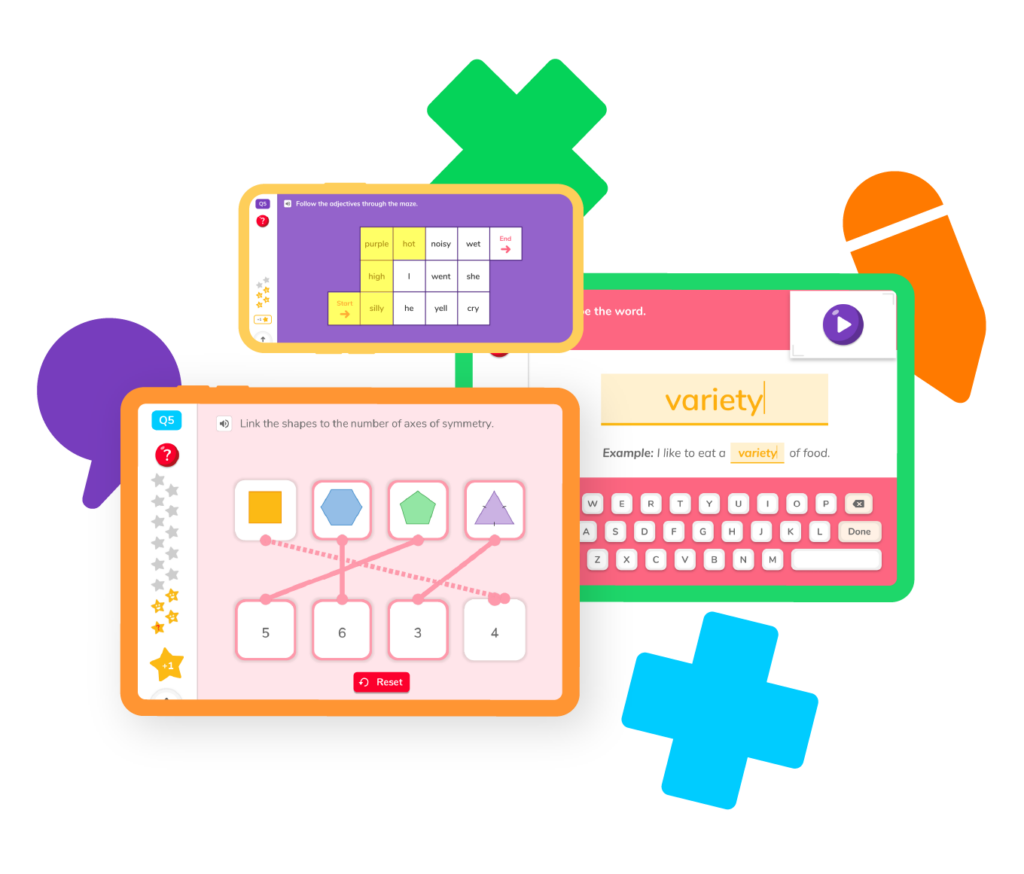More, more, more! Addition is all about making more of something, whether it’s making a number bigger or giving someone more of what they need or want. It’s also a crucial skill to understand if you hope to become a math expert. With a little bit of practice and a whole lot of determination, you’ll get addition down in no time!Like subtraction, addition starts simple but gets more involved as you climb through 1st, 2nd, and 3rd grade. You can learn different skills to solve your addition problems with ease — and prepare for addition in the real world!  Let’s take a closer look at the fun things you can do with addition.

## Add with shapes and props

Use blocks, apples, and more to add two quantities to create a new sum.

## Find the sum

Learn how to add two numbers together to find the sum.

Explore different methods of adding two-digit numbers together.

Discover how to add two fractions together.

Practice finding the sum of three and four-digit numbers.

## Add with shapes and props

Use blocks, apples, and more to add two quantities to create a new sum.

## Find the sum

Learn how to add two numbers together to find the sum.

Explore different methods of adding two-digit numbers together.

Discover how to add two fractions together.

Practice finding the sum of three and four-digit numbers.While addition is one of the most basic concepts in mathematics, it may be tough to get the hang of it at first. Just know you are not alone. Here are some of the more frequently asked questions about addition.

### What terms do I need to know to understand addition?

There aren’t as many terms you will need to know to understand addition as there are to understand subtraction. You need to know two basic terms to understand the definition of addition:

• Addend – One of the two numbers you are adding together
• Sum – What you get when you add the addends together

### What are common strategies for solving addition problems?

There are plenty of strategies you can use to solve addition problems. Some of the most common include using a number line, counting on your fingers, visualizing the problem (turning each addend into a picture so you can ‘see’ how many you have when you put them together), and more. Explore these strategies in the Skills section above.

### When do students begin to learn addition?

You will begin to practice addition in kindergarten. You might receive three blocks, and your teacher asks you to take two more blocks. How many blocks do you have now? You will start to practice addition problems in first grade, then get into more advanced problems in 2nd and 3rd grades.

### Can I add fractions together?

Yes, you can add fractions together. You’ll likely tackle these types of problems in third or fourth grade. Your teacher will show you how to add fractions with like and unlike denominators. When adding fractions with unlike denominators, you’ll need to make sure the denominators are the same. This means you will have to understand multiplication to successfully add fractions. For example, if you want to add 1/3 and 2/5 together, you will need to know that 3 x 5 = 15, and then multiply the top numbers by 5 and 3, respectively.

This will leave you with 5/15 and 6/15, which you can add together to get 11/15.

### Will I use addition in real life?

All the time! You will use addition to predict how much money you will have after you get paid, how many items you will need for projects and hobbies, how many RSVPs you have received for a birthday party, and so much more! It’s one of the most important skills you can learn.# Are you a parent, teacher or student?

Are you a parent or teacher?

## Hi there!

Book a chat with our team